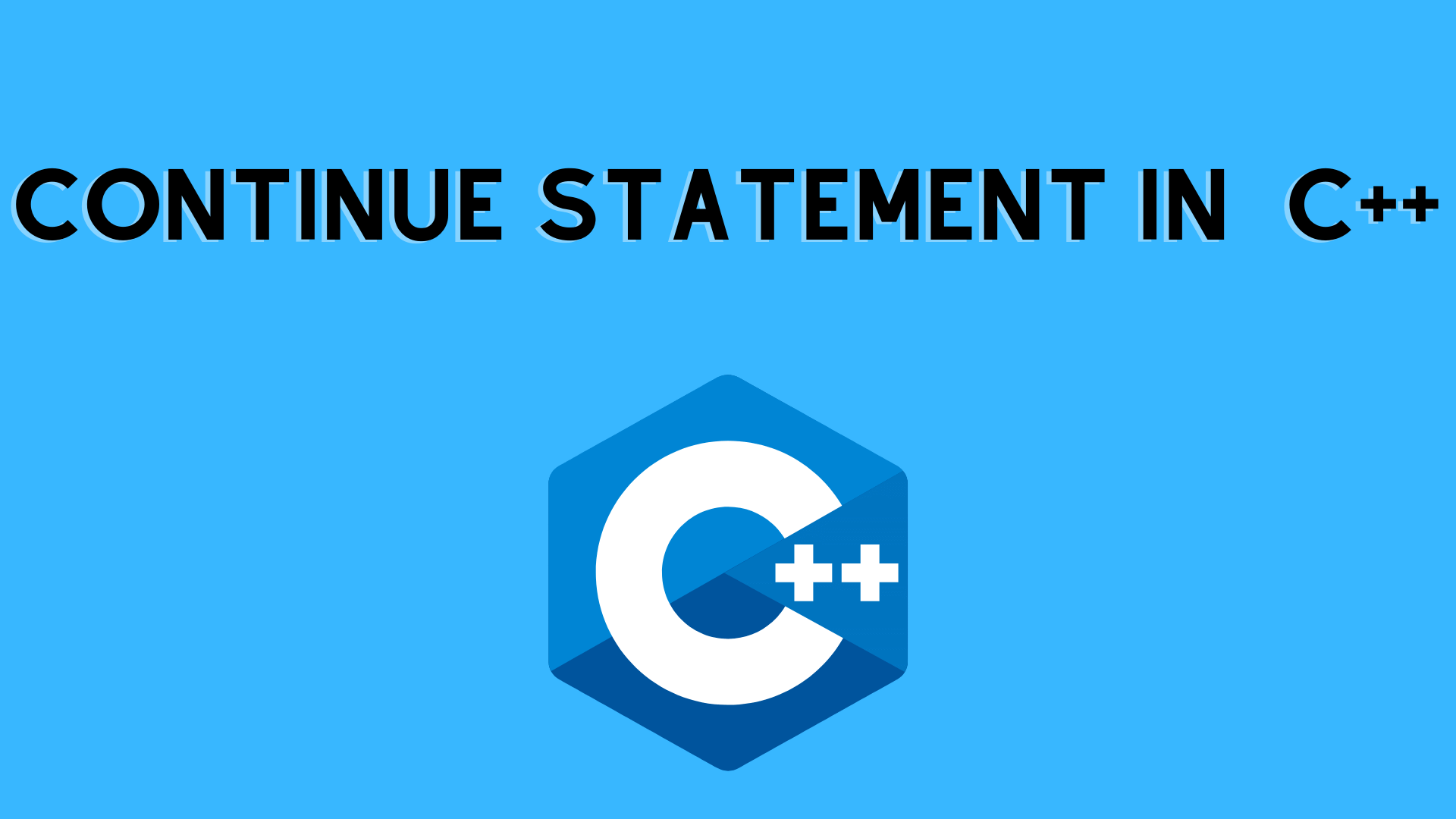## C++ Continue Statement with Example | C++ Programming

In the previous tutorial, we have learned about the Break statement. In this, we will learn about the Continue statement with an example.

## Definition of Continue statement

The continue statement is used inside loops. When a continue statement is found within a loop, control is returned to the beginning of the loop for the next iteration, skipping the execution of statements within the loop’s body for the current iteration.

• When you need to jump to the next iteration of the loop right away, you utilize the continue statement in the loop control structure. It can be used in conjunction with a for loop or a while loop.
• The loop is continued using the C++ continue command. It continues the program’s current flow while skipping the remaining code at the specified circumstance. It only continues the inner loop in the case of an inner loop.

The continue statement in C++ can be used in all forms of loops, including for loopswhile loops, and do-while loops.

Syntax:

When working with loops, you may want to skip some statements or end the loop. Break and continue statements are used in such cases.

Example: Continue statement in For loop

```#include <iostream>
using namespace std;

int main() {
for (int i = 1; i <= 5; i++) {
// condition to continue
if (i == 3) {
continue;
}

cout << i << endl;
}

return 0;
}```

Output

1
2
4
5

Continue skips the current iteration in a for loop and directs the control flow to the update expression.

```if (i == 3) {
continue;
}```

These Means

• The continue statement skips the current iteration and starts the next one when I equal 3.
• The condition is then re-evaluated when I become 4.
• As a result, the next two iterations print 4 and 5.

Example: Continue in While Loop

```#include <iostream>
using namespace std;

int main() {
int sum = 0;
int number = 0;

while (number >= 0) {
sum += number;

// take input from the user
cout << "Enter a number: ";
cin >> number;

// continue condition
if (number > 50) {
cout << "The number is greater than 50 and won't be calculated." << endl;
number = 0;  // the value of number is made 0 again
continue;
}
}

// display the sum
cout << "The sum is " << sum << endl;

return 0;
}```

Output

Enter a number: 12
Enter a number: 0
Enter a number: 2
Enter a number: 30
Enter a number: 50
Enter a number: 56
The number is greater than 50 and won’t be calculated.
Enter a number: 5
Enter a number: -3
The sum is 99

Continue skips the current iteration in a while loop, and the program’s control flow returns to the while condition.

```if (number > 50){
continue;
}```

Example: Continue Statement in do-while Loop

```#include <iostream>
using namespace std;

int main() {
int i=1;
do{
if(i==5){
i++;
//using continue statement
continue;
}
cout<<i<<endl;
i++;
}while(i<=10);

return 0;
}```

Output:

Example:- Continue in Nested loop

When the continue statement is used with nested loops, the current iteration of the inner loop is skipped. As an example:

```#include <iostream>
using namespace std;

int main() {
int number;
int sum = 0;

// nested for loops

// first loop
for (int i = 1; i <= 3; i++) {
// second loop
for (int j = 1; j <= 3; j++) {
if (j == 2) {
continue;
}
cout << "i = " << i << ", j = " << j << endl;
}
}

return 0;
}```

Output

i = 1, j = 1
i = 1, j = 3
i = 2, j = 1
i = 2, j = 3
i = 3, j = 1
i = 3, j = 3

When the continue statement is used in the above program, it skips the current iteration of the inner loop. And the program’s control shifts to the inner loop’s update expression.

As a result, the value of j = 2 never appears in the output.

Summary:

Within loops, the continue statements are commonly used. Control jumps to the beginning of the loop for the next iteration when it is encountered within a loop, skipping the execution of statements within the loop’s body for the current iteration. This is particularly useful when you want to continue the loop but don’t want the remaining statements in the loop body ( after the continue statements) to run for that iteration.